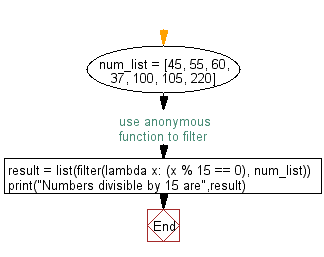﻿ Python: Get numbers divisible by fifteen from a list using an anonymous function - w3resource# Python: Get numbers divisible by fifteen from a list using an anonymous function

## Python Basic: Exercise-110 with Solution

Write a Python program to get numbers divisible by fifteen from a list using an anonymous function.

Sample Solution-1:

Python Code:

``````num_list = [45, 55, 60, 37, 100, 105, 220]
# use anonymous function to filter
result = list(filter(lambda x: (x % 15 == 0), num_list))
print("Numbers divisible by 15 are",result)
```
```

Sample Output:

```Numbers divisible by 15 are [45, 60, 105]
```

Flowchart:## Visualize Python code execution:

The following tool visualize what the computer is doing step-by-step as it executes the said program:

Sample Solution-2:

Python Code:

``````num_list = [45, 55, 60, 37, 100, 105, 220]
print("Original list:",num_list)
# use anonymous function to filter
result = list(filter(lambda x: (x % 15 == 0), num_list))
print("Numbers of the said list divisible by 15 are:",result)
```
```

Sample Output:

```Original list: [45, 55, 60, 37, 100, 105, 220]
Numbers of the said list divisible by 15 are: [45, 60, 105]
```

## Visualize Python code execution:

The following tool visualize what the computer is doing step-by-step as it executes the said program:

Sample Solution-3:

Python Code:

``````num_list = [45, 55, 60, 37, 100, 105, 220]
print("Original list:",num_list)
print("\nNumbers of the said list divisible by 15 are:")
print(str(''.join(filter(lambda x: x, str(list([i for i in num_list if i % 15 == 0]))))))
```
```

Sample Output:

```Original list: [45, 55, 60, 37, 100, 105, 220]

Numbers of the said list divisible by 15 are:
[45, 60, 105]
```

## Visualize Python code execution:

The following tool visualize what the computer is doing step-by-step as it executes the said program:

Python Code Editor:

Have another way to solve this solution? Contribute your code (and comments) through Disqus.

What is the difficulty level of this exercise?

Test your Python skills with w3resource's quiz

﻿

## Python: Tips of the Day

Unpack variables from iterable:

```# One can unpack all iterables (tuples, list etc)
>>> a, b, c = 1, 2, 3
>>> a, b, c
(1, 2, 3)

>>> a, b, c = [1, 2, 3]
>>> a, b, c
(1, 2, 3)
```